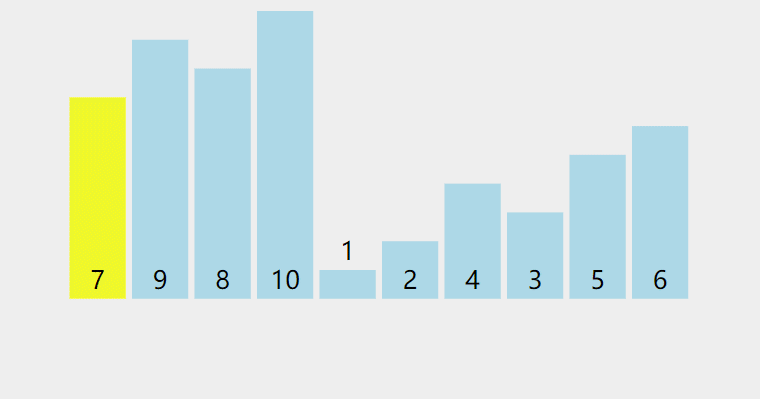### 排序思路

1. 从数列中挑出一个元素，称为「基准」（pivot），
2. 重新排序数列，所有比基准值小的元素摆放在基准前面，所有比基准值大的元素摆在基准后面（相同的数可以到任何一边）。在这个分割结束之后，该基准就处于数列的中间位置。这个称为分割（partition）操作。
3. 递归地（recursively）把小于基准值元素的子数列和大于基准值元素的子数列排序。
递归到最底部时，数列的大小是零或一，也就是已经排序好了。这个演算法一定会结束，因为在每次的迭代（iteration）中，它至少会把一个元素摆到它最后的位置去。

$$f(R,s,t) \begin{cases} nothing \qquad \text {R[s..t]中没有或者只有一个元素} \\ \\ do \begin{cases} i =partiton(R,s,t);\\f(R,s,i-1); \qquad \text {其他情况} \\f(R,i+1,t); \end{cases} \end{cases}$$

### 排序算法

#include<stdio.h>

int partition(int R[],int s,int t){
int i=s,j=t;//这里取第一个元素为基准，你也可以取其他元素
int tmp=R[i];
while(i<j){
while(i<j&&R[j]>=tmp)j--;//从右边取第一个比它小的元素，放到左边
R[i]=R[j];
while(i<j&&R[i]<=tmp)i++;//从左边取第一个比它大的元素，放到右边
R[j]=R[i];
}
R[i]=tmp;//一次分割完成，右边的元素<=R[i]<=左边的元素，全局有序

//打印结果
int k;
for (k = 0; k < 10;k++){
printf("%d ",R[k]);
}
printf("\n");

return i;
}
void quick_sort(int R[],int s,int t){
int i;
if(s<t){
i=partition(R,s,t);
quick_sort(R,s,i-1);
quick_sort(R,i+1,t);
}
}
int main(void){
int a[] = {6,8,7,9,0,1,3,2,4,5};
quick_sort(a,0,9);
}

5 4 2 3 0 1 6 9 7 8
1 4 2 3 0 5 6 9 7 8
0 1 2 3 4 5 6 9 7 8
0 1 2 3 4 5 6 9 7 8
0 1 2 3 4 5 6 9 7 8
0 1 2 3 4 5 6 8 7 9
0 1 2 3 4 5 6 7 8 9
##### 过程分析

6 8 7 9 0 1 3 2 4 5  为例，

• * 8 7 9 0 1 3 2 4 5 从右边开始遍历，寻找第一个比6小的元素。找到5，将5放到6原来的位置。
• 5 8 7 9 0 1 3 2 4 * 从左边开始遍历，寻找第一个比6大的元素。找到8，将8放到5原来的位置。
• 5 * 7 9 0 1 3 2 4 8
• ......
• 5 4 2 3 0 1 * 9 7 8 直到i==j ，将tmp=6放回，一次分割完成。

#include<stdio.h>

int partition(int R[],int s,int t){
int tmp=R[s];//这里选择s为基准，也可以选择其他元素
R[s]=R[t];//这基准元素，放到最后一个位置
R[t] = tmp;
int i,index = s;//这里记录下分割位置
for (i = s; i < t;i++){//从第一个遍历至倒数第二个,找到其位置
if(R[i]<tmp){//tmp较大,tmp位置应该滞后
int tmp2 = R[index];//交换index和当前元素
R[index] = R[i];
R[i] = tmp2;
index++;//滞后
}
}
int tmp2 = R[index];// 将基准元素放入
R[index] = R[t];
R[t] = tmp2;

//打印结果
int k;
for (k = 0; k < 10;k++){
printf("%d ",R[k]);
}
printf("\n");
return index;
}
void quick_sort(int R[],int s,int t){
int i;
if(s<t){

i=partition(R,s,t);
quick_sort(R,s,i-1);
quick_sort(R,i+1,t);
}
}
int main(void){
int a[] = {6,8,7,9,0,1,3,2,4,5};
quick_sort(a,0,9);
}### 算法分析

##### 时间复杂度、空间复杂度：
1. 平均情况：

$$执行时间 \quad T(n) = cn+\frac{1}{n}\sum_{k=1}^{n}(T(k-1)+T(n-k)) \qquad其中一次分割为线性时间，不妨设为cn$$

1. 最坏情况：递归树高度为n，每一层划分时间为n-1，所以时间复杂度为O(n^2^) ，空间复杂度为O(n)
2. 最好情况：递归树高度为O(log~2~n)，每一层划分时间为O(n)，所以时间复杂度为O(nlog~2~n) ，空间复杂度为O(log~2~n)

##### 归位：

Responses
1.hello

study 一起学习 go